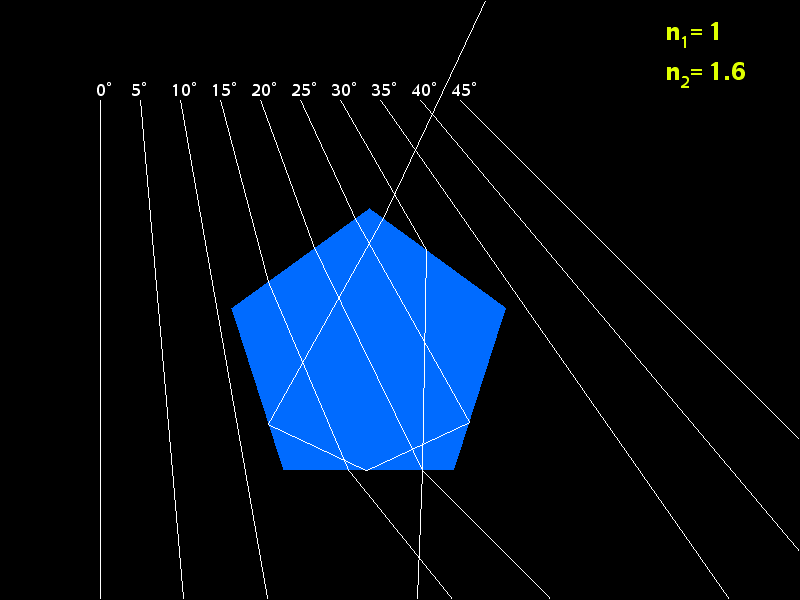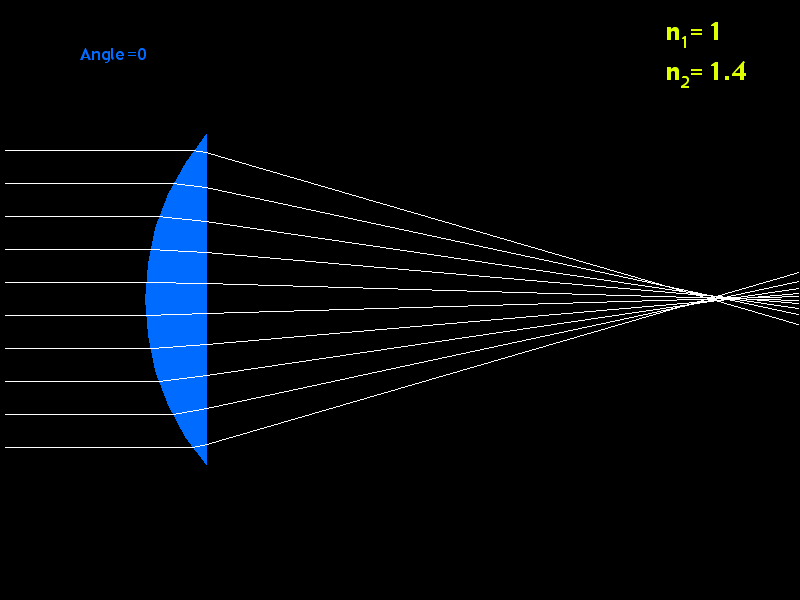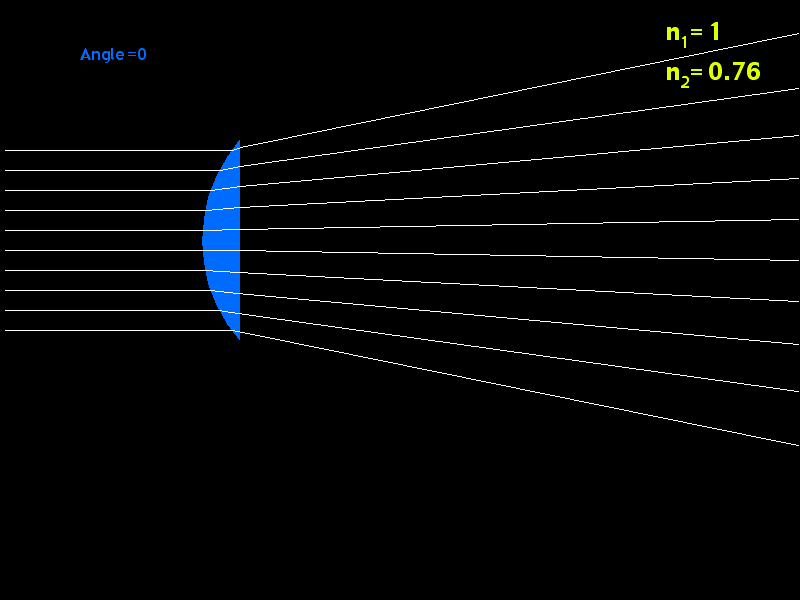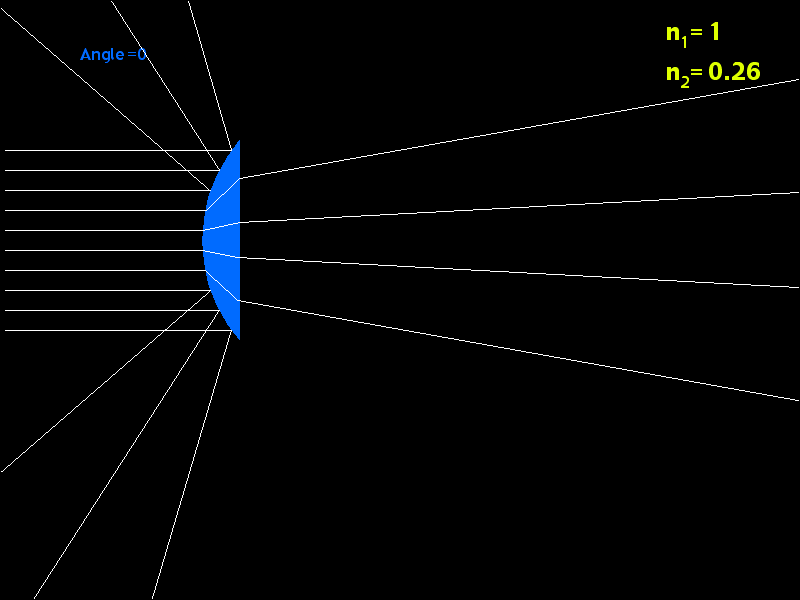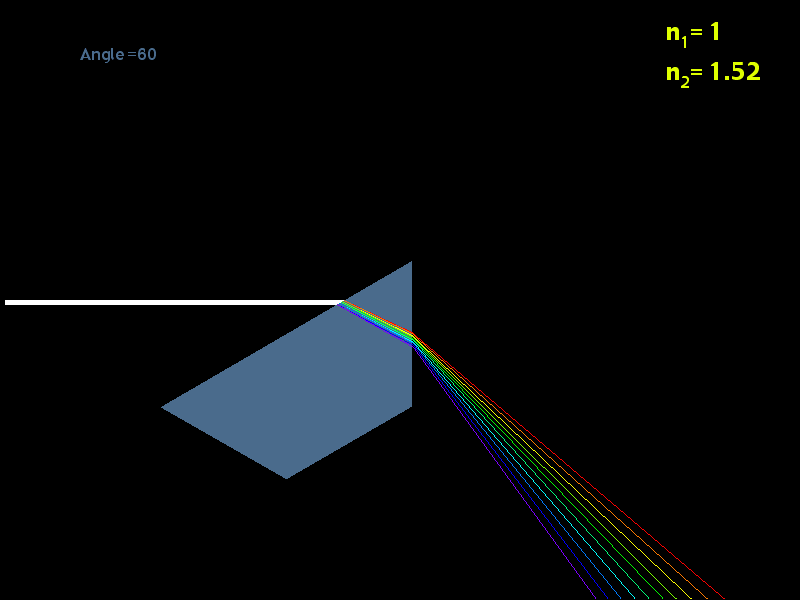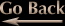Here are some images I made as programming exercise. Given the angle of incidence and the index of refraction of a particular medium, calculate the surface normal and angle of refraction (or if refraction is theoretically impossible at the angle of incidence, calculate the angle of reflection)

Scroll down for more images